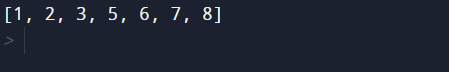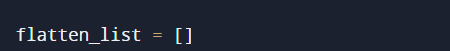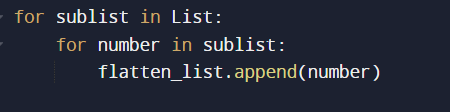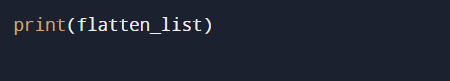# Python Program to Make a Flattened List from Nested List

In this tutorial you will learn about the Python Program to Make a Flattened List from Nested List and its application with practical example.

In this tutorial, we will learn to create a Python Program to Make a Flattened List from Nested List using python Programming.

## Prerequisites

Before starting with this tutorial we assume that you are best aware of the following Python programming topics:

• Python Operator.
• Basic Input and Output
• Basic Python  programming.
• Python if-else statements.
• Python Loop.
• List in Python.

## How do you flatten a list in Python?

Flattening a list refers to the process of removing a dimension from a list.
There are three ways to flatten a list:

1. Using a list comprehension.
2. By Using a nested for loop.
3. Using the ‘itertools’ method chain().

## Python Program to Make a Flattened List from Nested List

In this program we will create a program to make a Flattened List from Nested List .First we would declared and initialized the required variables.then we make a Flattened List from Nested List.

## Output

Flatten_list.In our Python Program to Make a Flattened List from Nested List. First we would declared and initialized the required variables. Next, we would prompt user to input the value  then Flattened List from Nested  List.

In our program, we have first declared and initialized a set variables required in the program.

• List = Declaring list in it.
• flatten_list= it will show flatten list .
• sublist=it will hold temporary list.
• number= it will hold list number.Then we create an empty list flatten_list.Now we are going to access each and all element’s of list in the “sublist” using a nested loop and  append the element in to flatten_list variable.In this tutorial will learnt to make a flattened list from a nested list and at last we will print the flatten list to see our output.In this tutorial we have learn about the Python Program to Make a Flattened List from Nested List and its application with practical example. I hope you will like this tutorial.